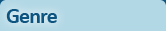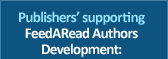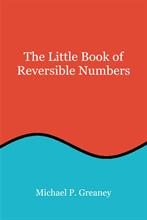# The Little Book of Reversible NumbersReversible numbers are pairs of numbers in which the digits (or numerals) of one number are in the reverse order to those of the other, e.g., 39 and 93. These include palindromic numbers in which read the same forwards as backwards, e.g., 747. The fun starts when the various operations of addition, subtraction, multiplication and division are carried out between pairs of such numbers. This book begins with a look at the unexpected way in which addition and subtraction can be carried out with reversible numbers, a way that cannot be done with non-reversible pairs. It then looks at how the subtraction between reversible pairs leads to an equation that shows the relationship between a number and its reverse, that they are not just two arbitrary, unrelated numbers. Some simple equations for addition and subtraction are derived and are shown to be useful for analysis. Some interesting behaviour occurs when repetitive addition and subtraction are carried out, using the sum or difference as the new number. The Kaprekar Operation takes the difference between a reversible pair and reorders the digits in descending order with each iteration. Lychrel numbers are reversible pairs which are added together. The digits are not reordered; the repetitive addition is left to run. Eriam numbers are like Lychrel numbers but are subtracted instead of added. The outcome, though, is quite different. The book also looks what happens and what does not when multiplying and dividing reversible numbers. It is a subject that lends itself to exploration by computer and hints are given throughout the book on how this can be done. It is not a book on computing, however. It will appeal to people who enjoy the number patterns that are produced by performing different operations on reversible pairs of numbers. Some useful tools to enable readers to explore this subject for themselves are presented in the appendices, along with web links to various sites for those who wish to go deeper into the subject.
ISBN: 9781839459160
Type: Paperback
Pages: 132
Published: 23 April 2021
Price: \$10.25